# einsum初探

### Einsum 是干嘛的？

A = np.array([0, 1, 2])
B = np.array([[ 0,  1,  2,  3],
[ 4,  5,  6,  7],
[ 8,  9, 10, 11]])

(A[:, np.newaxis] * B).sum(axis=1)
array([ 0, 22, 76])

np.einsum('i,ij->i', A, B)
array([ 0, 22, 76])

### 如何使用 einsum

np.einsum('ij,jk->ik', A, B)

A = np.array([[1, 1, 1],
[2, 2, 2],
[5, 5, 5]])
B = np.array([[0, 1, 0],
[1, 1, 0],
[1, 1, 1]])
np.einsum('ij,jk->ik', A, B)可以看作是：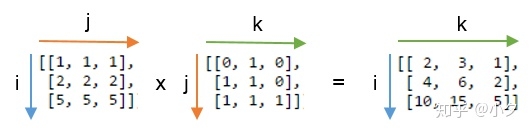• 在输入数组的标记之间，重复字母表示沿这些轴的值将相乘，这些乘积构成输出数组的值。比如图中沿着j轴做乘积。
• 从输出标记中省略的字母表示沿该轴的值将被求和。比如图中的输出没有包含j轴，因此沿着j轴求和得到了输出数组中的每一项。
• 如果输出的标记是'ijk'，那么会得到一个 3x3x3 的矩阵。
• 输出标记是'ik'的时候，并不会创建中间的 3x3x3 的矩阵，而是直接将总和累加到2D数组中。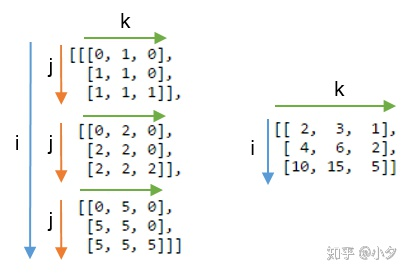• 如果输出的标记是空，那么输出整个矩阵的和。
• 我们可以按任意顺序返回不求和的轴。

'ij,jk->ik' 等价于 'ij,jk'

### Einsum 中的常用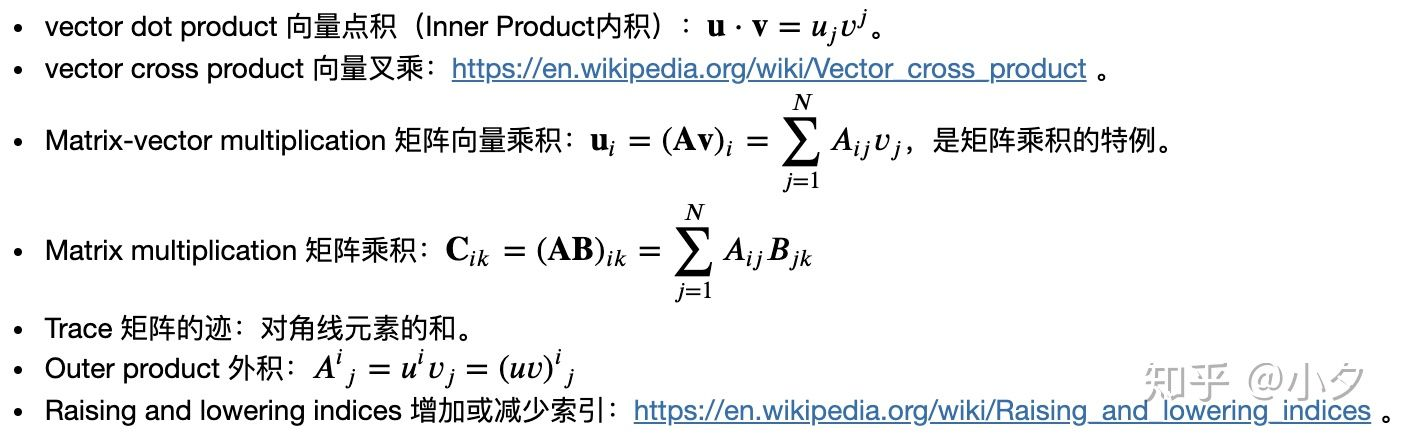### 对应的 einsum调用方式：

• 向量操作：A、B均为向量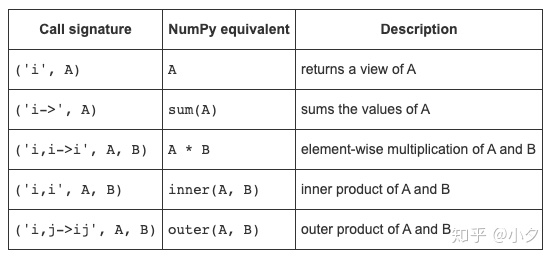• 向量操作：A、B均为2D矩阵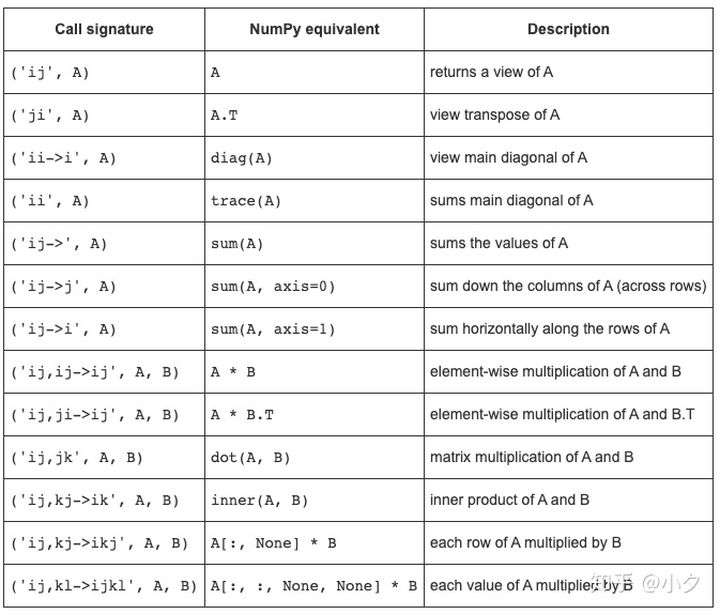### 注意

• einsum求和时不提升数据类型，如果使用的数据类型范围有限，可能会得到意外的错误：
a = np.ones(300, dtype=np.int8)
print(np.sum(a)) # correct result
print(np.einsum('i->', a)) # produces incorrect result
300
44
• einsum 在implicit mode可能不会按预期的顺序排列轴
M = np.arange(24).reshape(2,3,4)
print(np.einsum('kij', M).shape) # 不是预期
print(np.einsum('ijk->kij', M).shape) #符合预期
(3, 4, 2)
(4, 2, 3)
np.einsum('kij', M) 实际上等价于 np.einsum('kij->ijk', M)，因为 implicit mode 下，einsum会认为根据输入标记，按照字母表顺序排序，作为输出标记。
• 最后，einsum 也不总是numpy中的最快的选择。
dot和inner函数之类的功能通常会链接到BLAS库方法，性能可能胜过einsum。 还有tensordot函数。 在多个输入数组上进行操作时，einsum似乎很慢。

------------------------------ 华丽丽的分割线 ------------------------------

### numpy 中的 einsum

import numpy as np
# 给定两个向量，下面要用到
a = np.array([1, 2, 3])
b = np.array([4, 5, 6])
# 给定两个矩阵，下面要用到
A = np.array([[1, 2], [3, 4]])
B = np.array([[5, 6], [7, 8]])

### 接口定义：

numpy.einsum(subscripts, *operands, out=None, dtype=None, order='K', casting='safe', optimize=False)

### 参数:

xxx表示可选参数中的默认值）

• subscripts : str，指定求和的操作，可以是多个，用逗号分开。除非包含显式指示符“->”以及精确输出形式的下标标签，否则将执行隐式（经典的爱因斯坦求和）计算。
• operands : list of array_like，输入。
• out : ndarray, optional，指定输出。
• dtype : {data-type, None}， optional，可以指定运算的数据类型，可能需要用户提供类型转换接口。默认为None。
• order : {‘C’, ‘F’, ‘A’, ‘K’}， optional，控制输出的内存布局。'C'=contiguous；'F'=Fortran contiguous；'A'表示输入为'F'时输出为'F'，否则输出为'C'；'K'表示输出的layout应该尽可能和输入一致。
• casting : {‘no’, ‘equiv’, ‘safe’, ‘same_kind’, ‘unsafe’}， optional，指定可能发生的数据类型转换，不推荐使用'unsafe'。
• ‘no’ 表示不做数据类型转换。
• ‘equiv’ 表示仅允许字节顺序更改。
• ‘safe’ 表示只允许保留值的强制类型转换。
• ‘same_kind’ 表示仅允许安全类型转换或同一类型（例如float64到float32）内的类型转换。
• ‘unsafe’ 表示可以进行任何数据转换。
• optimize : {False, True, ‘greedy’, ‘optimal’}, optional，控制是否进行中间优化。默认False，不做优化；设为True则使用greedy算法。还接受np.einsum_path函数的提供的列表。

### Returns:

• output : ndarray

np.einsum('ij,jh', A, B) # 返回的是矩阵乘的转置，因为'h'本来应该是在'i'的后面，但是这里反序了
array([[19, 43],
[22, 50]])

np.einsum('ij,jh->ih', A, B) # 指定了输出下标标签的顺序，因此效果等价于矩阵乘法 np.matmul(A,B)
# 相比之下，implicit 模式的 np.einsum('ij,jh', A, B) 效果等价于矩阵乘的转置
array([[19, 43],
[22, 50]])

np.einsum(A, [0,0])
5

optimize参数优化 einsum 表达式的收缩顺序，对于具有三个或更多操作数的收缩，这可以大大增加计算效率，但需要在计算过程中增加内存占用量。

1. 求矩阵的迹：
print(np.einsum('ii', A)) # implicit mode
print(np.einsum(A, [0,0]))# einsum(op0, sublist0, op1, sublist1, ..., [sublistout])方式 implicit mode
print(np.trace(A))
5
5
5
1. 矩阵的对角元素
print(np.einsum('ii->i', A)) # explicit mode
print(np.einsum(A, [0,0], ))# einsum(op0, sublist0, op1, sublist1, ..., [sublistout])方式 explicit mode
print(np.diag(A))
[1 4]
[1 4]
[1 4]
1. 对指定维度求和
print(np.einsum('ij->i', A)) # explicit mode
print(np.einsum(A, [0,1], ))# einsum(op0, sublist0, op1, sublist1, ..., [sublistout])方式 explicit mode
print(np.sum(A, axis=1))
[3 7]
[3 7]
[3 7]

print(np.einsum('...j->...', A)) # explicit mode
print(np.einsum(A, [Ellipsis,1], [Ellipsis])) # einsum(op0, sublist0, op1, sublist1, ..., [sublistout])方式 explicit mode
[3 7]
[3 7]
1. 计算矩阵转置，或根据指定的 axes 调整矩阵
print(np.einsum('ji', A)) # implicit mode
print(np.einsum('ij->ji', A))# explicit mode
print(np.einsum(A, [1,0])) # einsum(op0, sublist0, op1, sublist1, ..., [sublistout])方式 implicit mode
print(np.einsum(A, [1,0], [0,1])) # einsum(op0, sublist0, op1, sublist1, ..., [sublistout])方式 explicit mode
print(np.transpose(A, axes=[1,0]))
[[1 3]
[2 4]]
[[1 3]
[2 4]]
[[1 3]
[2 4]]
[[1 3]
[2 4]]
[[1 3]
[2 4]]
1. 向量内积
print(np.einsum('i,i', a, a)) # implicit mode
print(np.einsum(a, , b, ))# einsum(op0, sublist0, op1, sublist1, ..., [sublistout])方式 implicit mode
print(np.inner(a, a))
14
32
14
1. 矩阵向量乘积
k = np.array([1,2])
print(np.einsum('ij,j', A, k)) # implicit mode
print(np.einsum(A, [0,1], k, ))# einsum(op0, sublist0, op1, sublist1, ..., [sublistout])方式 implicit mode
print(np.einsum('ij,j->i', A, k)) # explicit mode
print(np.einsum('...j,j->...', A, k)) # explicit mode with broadcast
print(np.dot(A, k))
[ 5 11]
[ 5 11]
[ 5 11]
[ 5 11]
[ 5 11]
print(np.einsum('...,...', 3, A)) # implicit mode
print(np.einsum(',ij', 3, A))# explicit mode
print(np.einsum(3, [Ellipsis], A, [Ellipsis]))
print(np.multiply(3, A))
[[ 3  6]
[ 9 12]]
[[ 3  6]
[ 9 12]]
[[ 3  6]
[ 9 12]]
[[ 3  6]
[ 9 12]]
1. Tensor Contraction
m = np.arange(60.).reshape(3,4,5)
n = np.arange(24.).reshape(4,3,2)
print(np.einsum('ijk,jil->kl', m, n)) # explicit mode
print(np.einsum(a, [0,1,2], b, [1,0,3], [2,3])) # einsum(op0, sublist0, op1, sublist1, ..., [sublistout])方式 explicit mode
print(np.tensordot(a,b, axes=([1,0],[0,1]))) #
[[4400. 4730.]
[4532. 4874.]
[4664. 5018.]
[4796. 5162.]
[4928. 5306.]]
[[4400. 4730.]
[4532. 4874.]
[4664. 5018.]
[4796. 5162.]
[4928. 5306.]]
[[4400. 4730.]
[4532. 4874.]
[4664. 5018.]
[4796. 5162.]
[4928. 5306.]]
1. 链式数组操作: For more complicated contractions, speed ups might be achieved by repeatedly computing a ‘greedy’ path or pre-computing the ‘optimal’ path and repeatedly applying it, using an einsum_path insertion. Performance improvements can be particularly significant with larger arrays. 待补充

### TODO: Tensorflow 中的 einsum; Pytorch 中的 einsum

03-15729

#### numpy einsum12-05445

#### NumPy's einsum

02-223254

#### Python numpy输出省略号

12-103359

#### numpy数据不用省略号表示、取消科学记数法

10-08512

#### Numpy array输出中包含省略号

12-202020

#### np.einsum函数用法

04-117728

#### python numpy库中省略号...的一些用法

11-08827

#### numpy.einsum-学习笔记©️2020 CSDN 皮肤主题: 大白 设计师: CSDN官方博客点击重新获取扫码支付1.余额是钱包充值的虚拟货币，按照1:1的比例进行支付金额的抵扣。
2.余额无法直接购买下载，可以购买VIP、C币套餐、付费专栏及课程。余额充值